# Excel 2010 COUNT Functions – COUNT, COUNTA, COUNTIF and COUNTBLANK

COUNT function in Excel is different from the SUM function. They are most definitely not the same and have different purposes. COUNT function essentially counts numbers, and not adds, a given data in a cell. Also it counts only number data and not text data. You can choose a set of cells that contain number data and check how many of them have numbers.

## Excel 2010 COUNT Function

Purpose of COUNT function is to count number data and ignore text data. To count, choose a cell and enter the COUNT syntax: = COUNT (Range).The range is the cells of whom the counting needs to be done, for example cell A1:C6. If you enter any number data later in these cells, the counting cell will automatically update. So remember to keep the counting cell in a corner of the worksheet or away from the path of data rows and columns.

In the below screenshot, It’s only Counting Number of cell contain only Number, between B2 to B10.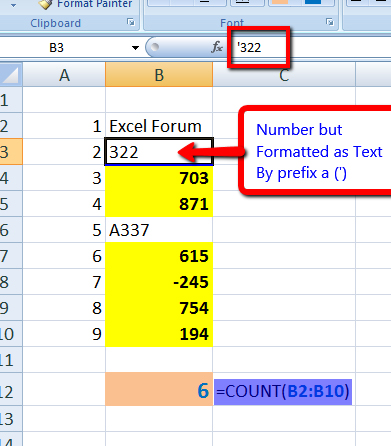In the same function, you add multiple Arguments also.

For example :

=COUNT(B2:B10,10,"10")

In above formula, two more arguments has been added, 10 & “10”.

However, excel will treat both arguments as Number, and count them.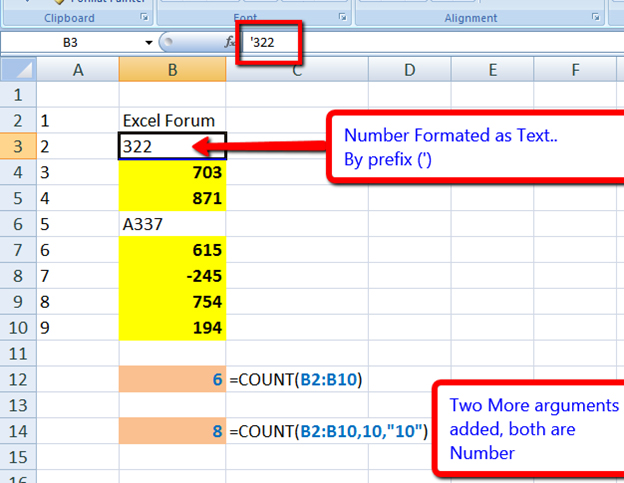## COUNTA function in Excel 2010

Counta function in excel is different from the count function. It's count all cells, but not blanks. COUNTA is usually not present in exel as default in AutoSum menu. Click on the More functions button and run a search for COUNTA. Easiest way is to create syntax directly as: =COUNTA (range) in the fx field.

In below example, it will only count the filled cell, even if cell is filled with a SPACE.

In second example, three more arguments has been added, but Excel will treat inserted all arguments as Countable.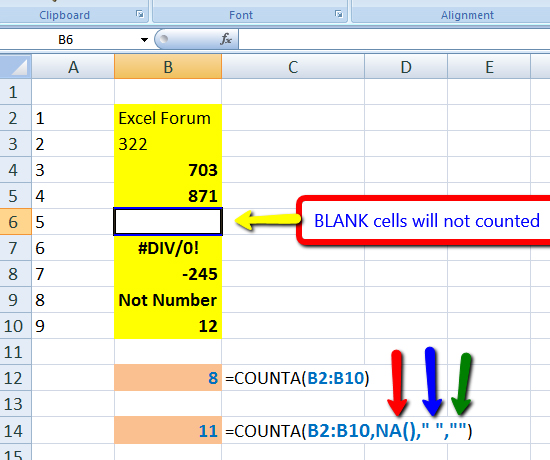# COUNTBLANK function in Excel 2010

As the name suggests, it counts the blank cells. In a massive data sheet, this is especially helpful to locate cells containing formula, along with empty cells.

If cell contain a Formula and formula return’s a BLANK, COUNTBLANK will count it as a blank.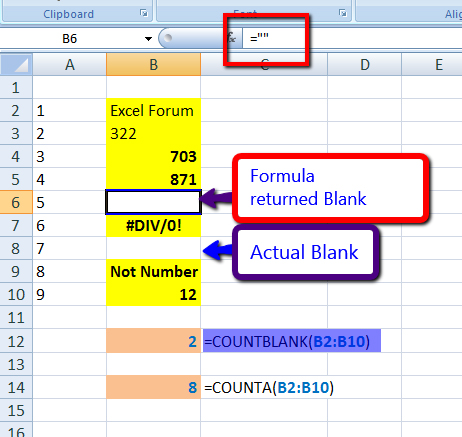However, COUNTA function will assume Formula Return Blank as a Non-Blank cell, and will Count it.

## COUNTIF and COUNTIFS function in Excel 2010

Counting cells that match certain criteria is done using COUNTIF and COUNTIFS functions. The first one is for a single range (example =COUNTIF(B2:B7,200)), where cells B2 to B7 will be searched for value equal to 200. For the second one, multiple ranges can be designated. (Example, =COUNTIFS(B2:B7,200,E2:E7,300)). Here B2 will be searched for ‘200’, while D1 to D6 will be searched for ‘300’. Only those cells that match these criteria will be counted. This is also called conditional counting.

You can also use this to count cells that contain “<” or “<=” (lesser than or equal to) or “>” or “>=” (greater than or equal to) values too.

Example :

=COUNTIFS (A1:A6,”>200”)

Or

=COUNTIFS (D1:D6,”<=300”)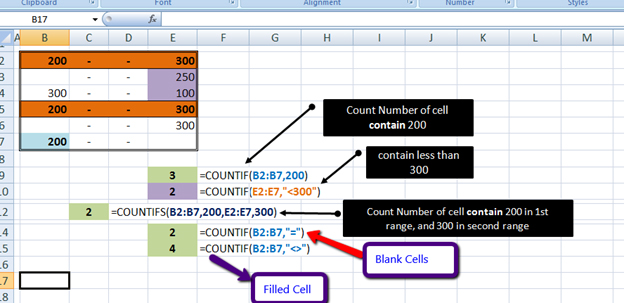These two functions can also be applied for text data. For example =COUNTIF (B1:B30, “*Biology*”) will count cells that contain the word ‘Biology’. The ‘*’ helps in counting uppercase and lower case form of the word. General syntax would be =COUNTIF(range, criteria).

If you need to count Number of Blank Cell in a range, you can use,

=COUNTIF(B2:B7,"=")

If you need to count Number of Filled Cell in a range, you can use,

=COUNTIF(B2:B7,"<>")

1.Is there a function that counts the hours and minutes between two times? Such as the number of hours between 9 am and 3 pm?

Thank you

2.Hi. I need help.
I am using COUNTA function to count all filled cells within the range. I need to exclude one particular value from that same count.
Thanks.

•Hey I think you are seeking for countif function which exclude one particular value as below

=+COUNTIF(range,"<>value")

•like for range e1 to e9 we have value,
7
7
5
6
7
8
6
9
a
and excluding 7 value from the range will use formula below

=COUNTIF(E1:E9,"<>7")

result = 6

•